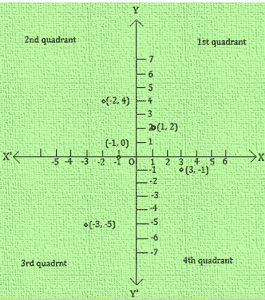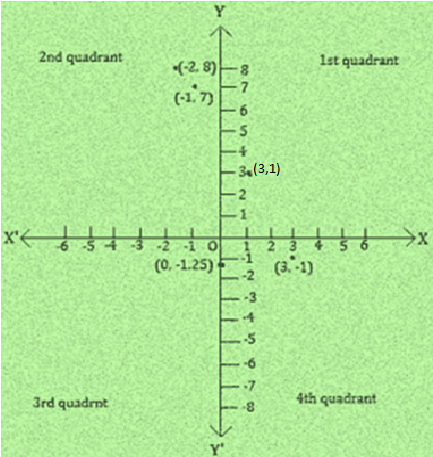# NCERT Solutions For Class 9 Math Chapter – 3 Exercise – 3.3

NCERT Solutions For Class 9 Math Chapter – 3 Exercise – 3.3

Q1.  In which quadrant or on which axis do each of the points (-2,4) (3,-1) (-1,0) (1,2) and (-3,-5) lie ? Verify your answer by locating them on the cartesian plane

Solution:-The  point (-2,4) is a having negative abscissa and positive ordinate. (-2,4) lies in the 2nd quadrant.

The point (3,-1) is having positive abscissa and negative ordinate. (-3,1) lies in the 4th quadrant.

The point (-1,0) is having negative abscissa and zero coordinate.

The point (-1,0) lies on the negative x – axis.

The point (1,2) having the abscissa as well as ordinate positive.

Point (1,2)  lies in the 1st quadrant.

The point (-3,5) is having the abscissa as well as ordinate negative.

Point (-3,-5) lies in the 3rd quadrant.

These points are plotted in the cartesian plane as shown in the figure as A(-2,4) B(3,-1) C(-1,0) D(1,2) E(-3,-5).

Q2. Plot the points (x,y) given in the following table on the plane , choosing suitable units of distance on the x – axis.

 x -2 -1 0 1 3 y 8 7 -1.25 3 -1

Solution:-

• We draw X’OX and YOY’ as axes.
• We choose suitable units of distances on the axes.
• To plot (-2,8) we start from O take (-2) units on x-axis and then (+8) units on y – axis. We mark th point as A(-2,8)
• To plot (-1,7) we start form O , take (-1) units on x – axis and then (+7) units on the y – axi. We mark the point as C(0,1.25).
• To plot (0.1.25) we move along 1.25 units below the x-axis on the y-axis and  mark the point as C(0.1.25)
• To plot (1,3) we take (+1) unit on the x-axis and then (+3) units on the y-axis We mark the point as D(1,3).
• To plot (3,-1) we take (+3) units on the x-axis and then (-1) unit on the y-axis We mark the point E(3,-1).